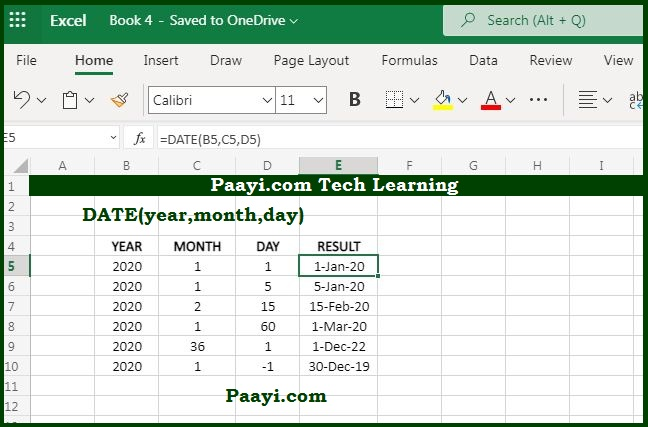# Learn How to Use Microsoft Excel DATE Function

Written by | 0 Comments | 697 Views

In this article, you will learn how to use the Microsoft Excel DATE function and its prime function in Microsoft Excel. You will also get to know the Microsoft Excel DATE function return value and syntax with the help of some examples.

## Microsoft Excel DATE Function

The Microsoft Excel DATE function's primary purpose is to create a date along with the year, month, and day. That means the DATE function makes a valid date from the specific year with year, month, and day as main components. The DATE function is used when you need to assemble dates that need to change dynamically as per the other values in the worksheet.

### DATE Function Return Value

It returns a valid excel date.

### DATE Function Syntax

= DATE(year, month, day)

Where:

• year: Number for the year
• month: Number for month
• day: Number for day

## How to Use Microsoft Excel DATE Function?As we know, the DATE function is used in the worksheet to create a valid excel date using individual year, month, and day. Let us understand how you can create the date:

• = DATE(1995, 1, 10)

This will create the date - January 10, 1995

• = DATE(2001,3,10)

This will create a date - March 10, 2001

The DATE function is also used for assembling dates that need to be changed dynamically as per other values, let's say 2020 is cell A1; let us see the example below:

• =Date(A1,1,10)
• Here the date is January 10, 2020, and when the A1 is changed to 2021, the date will be January 10, 2021.